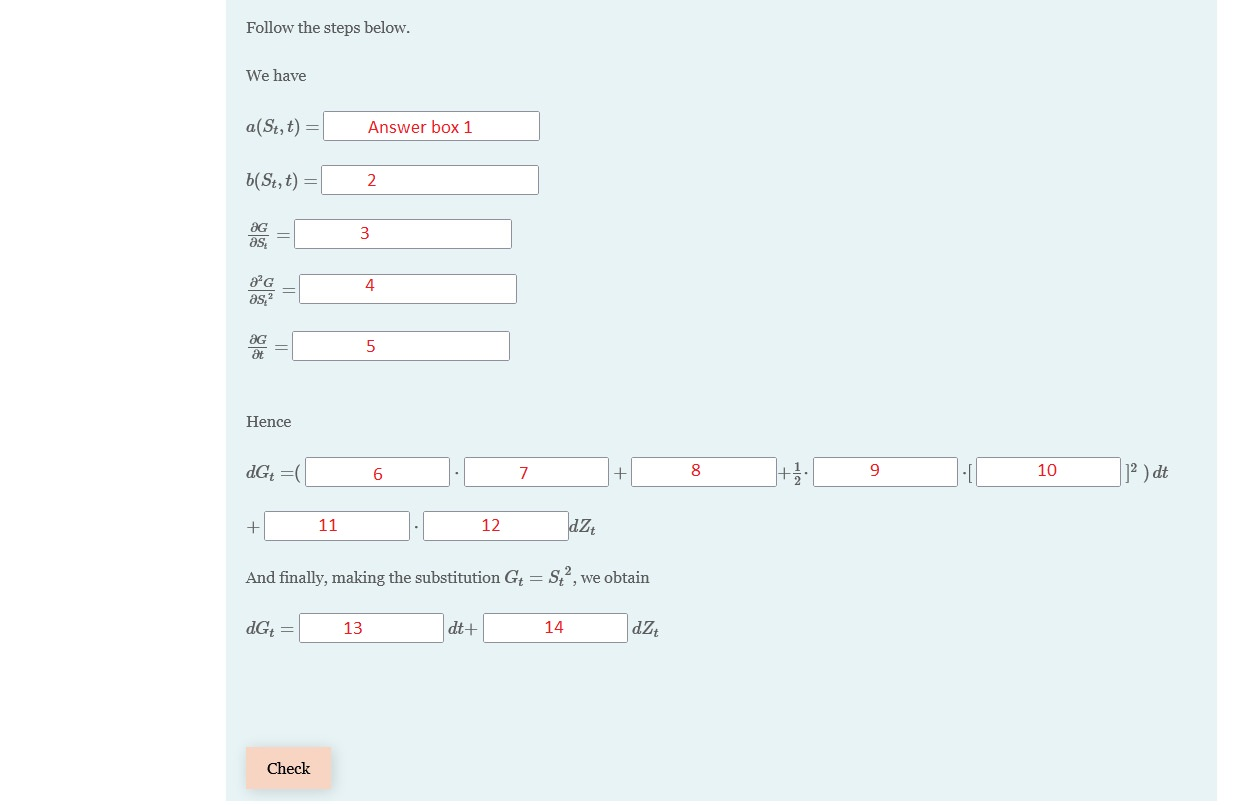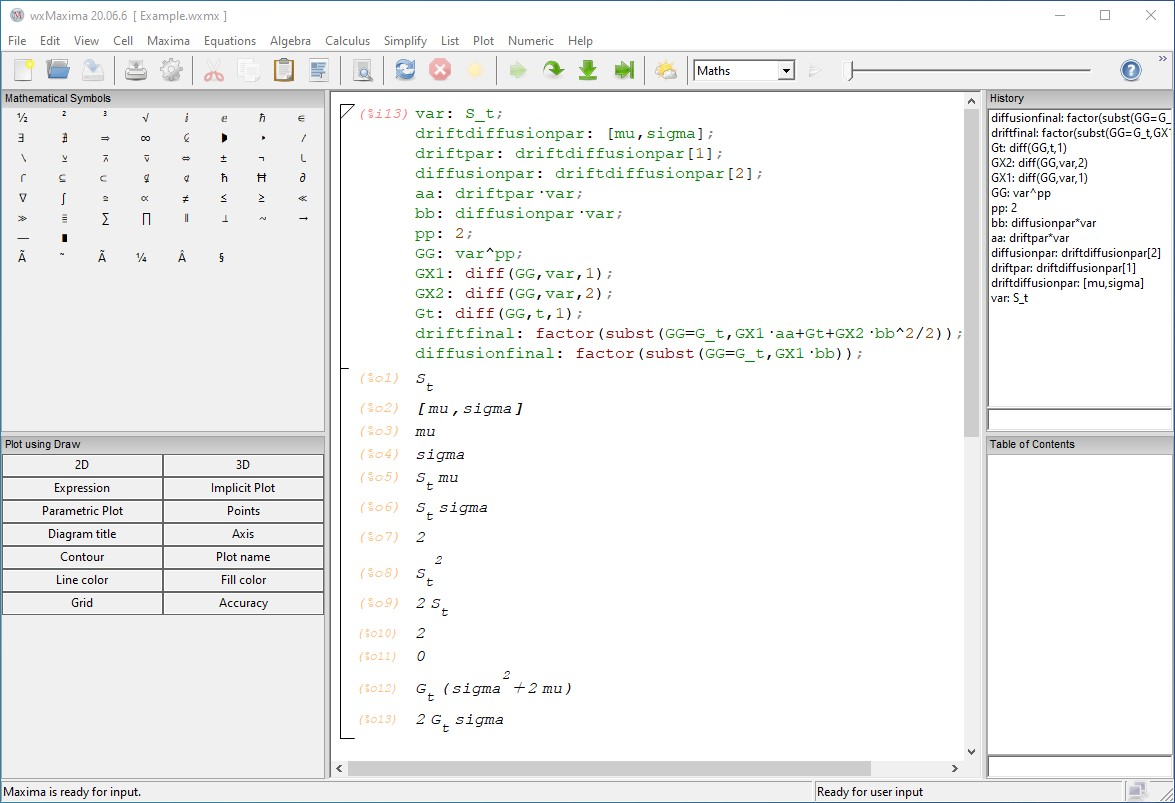### Outline

• Who is this talk primarily for?

• What are we going to do?

• What sort of things to look out for?

### Who is this talk primarily for?

Those of you who:

• use Moodle as a virtual learning environment or have a possibility to use it

• use STACK (question type for Moodle) or would like to use STACK

• would like to implement a longish exercise (with several answer boxes) in STACK; an example of a longish exercise is an application of Itô’s Lemma

Things that we will need:

• Moodle with STACK plug-in (you can use EMS2022 Moodle to practice)

• MAXIMA installation (MAXIMA is the computer algebra system behind STACK)

Things that will be helpful but not necessary:

• basic experience with/knowledge of STACK; if you don’t have it, no problem, you can follow ‘Authoring quick start’:

https://stack-demo.maths.ed.ac.uk/demo/question/type/stack/doc/doc.php/Authoring/Authoring_quick_start.md

• basic knowledge of MAXIMA

### What are we going to do?

• We will go over a longish exercise (Itô’s Lemma) in a general case

• We will go over a longish exercise (Itô’s Lemma) in a specific case, for which:

1. We will label the answer boxes from 1 to 14 (on a piece of paper)

2. Code it in MAXIMA

3. ‘Code’ it as a STACK question on Moodle

4. Randomize its sub-parts/elements (MAXIMA code)

5. Refine and finish

### Longish exercise (Itô’s Lemma) in a general case

Itô‘s Lemma: Given an Itô process $$\{X_t\}$$, $$dX_t=a(X_t,t) dt+ b(X_t,t) dZ_t$$, where $$\{Z_t\}$$ is the Wiener Process, $$a(\cdot,\cdot)$$ and $$b(\cdot,\cdot)$$ are functions of two variables satisfying some regularity conditions, the ’new’ Itô process $$\{G_t\}$$, $$G_t=G(X_t,t)$$, satisfies the following stochastic differential equation

$dG_t=\left( \frac{\partial G}{\partial X_t}a(X_t,t) + \frac{\partial G}{\partial t}+ \frac{1}{2} \frac{\partial^2 G}{\partial X_t^2} [b(X_t,t)]^2 \right) dt+ \frac{\partial G}{\partial X_t} b(X_t,t) dZ_t$

To apply this lemma we need to know how to:

1. Identify functions $$a(\cdot,\cdot)$$ and $$b(\cdot,\cdot)$$ in a given stochastic differential equation.

2. Find partial derivatives $$\frac{\partial G}{\partial X_t}$$, $$\frac{\partial^2 G}{\partial X_t^2}$$, $$\frac{\partial G}{\partial t}$$

3. Plug-in the elements from parts 1. and 2. into the formula from the lemma and then simplify the expression.

### Longish exercise (Itô’s Lemma) in a specific case

Let $$\{S_t\}$$ be an Itô process satisfying the following stochastic differential equation

$dS_t=\mu S_tdt+\sigma S_tdZt$ where $$\mu$$ and $$\sigma$$ are constants. ($$\{S_t\}$$ is called Geometric Brownian Motion.)

Apply Itô’s Lemma to derive a stochastic differential equation for the process $$\{G_t\}$$, where

$G_t=G(S_t,t)=S_t^2$

#### a) Label the answer boxes 1-14 (on a piece of paper)#### b) Code it in MAXIMA (screen-shot from MAXIMA)#### c) ‘Code’ it as a STACK question on Moodle (screen-shot from Moodle)

• In STACK’s $$\fbox{Question variables}$$ we insert MAXIMA code